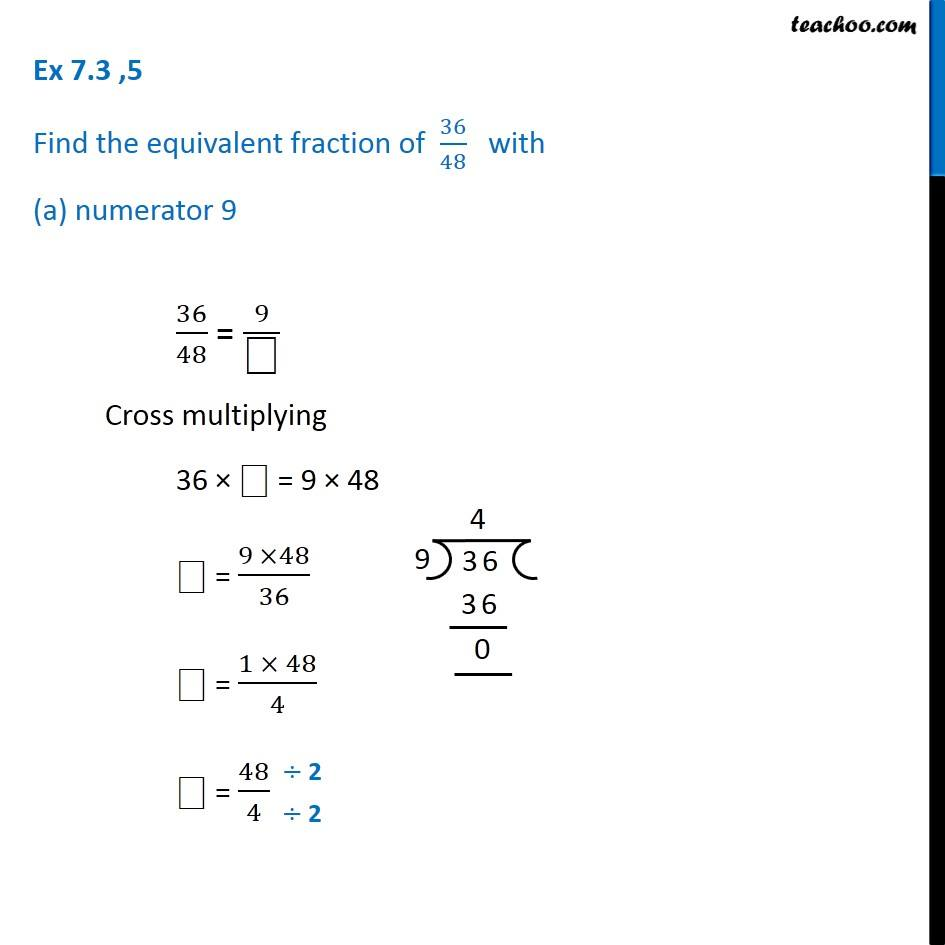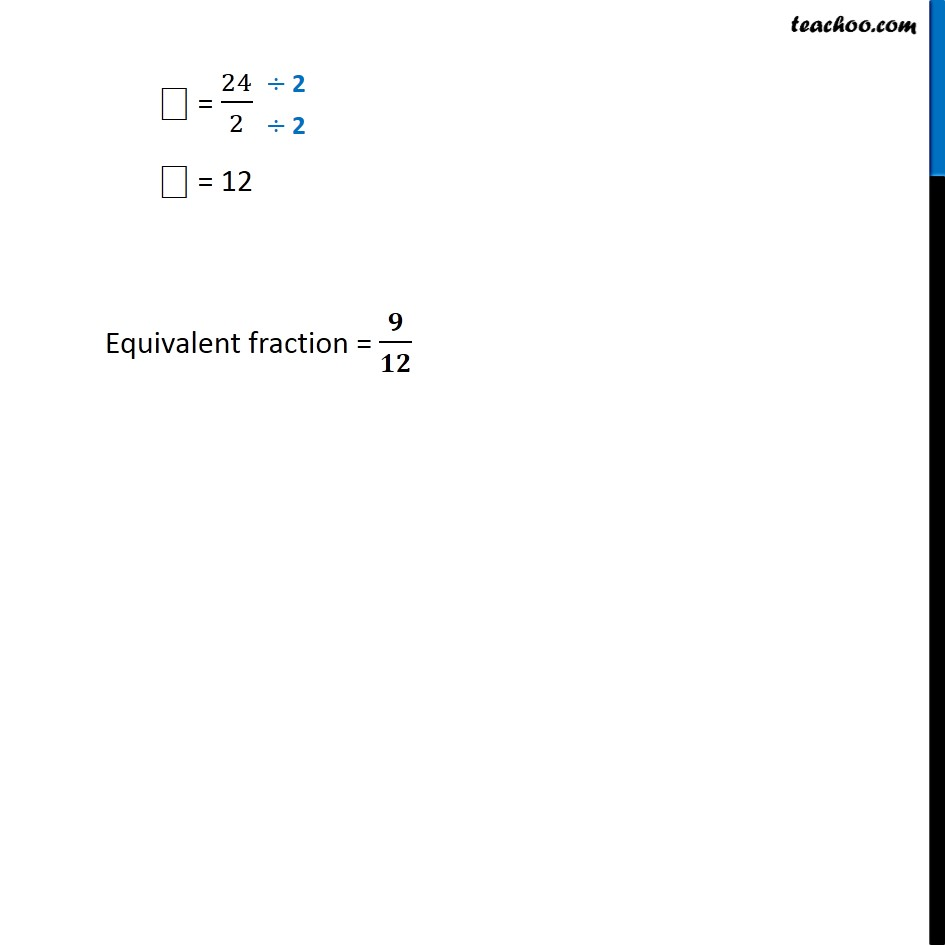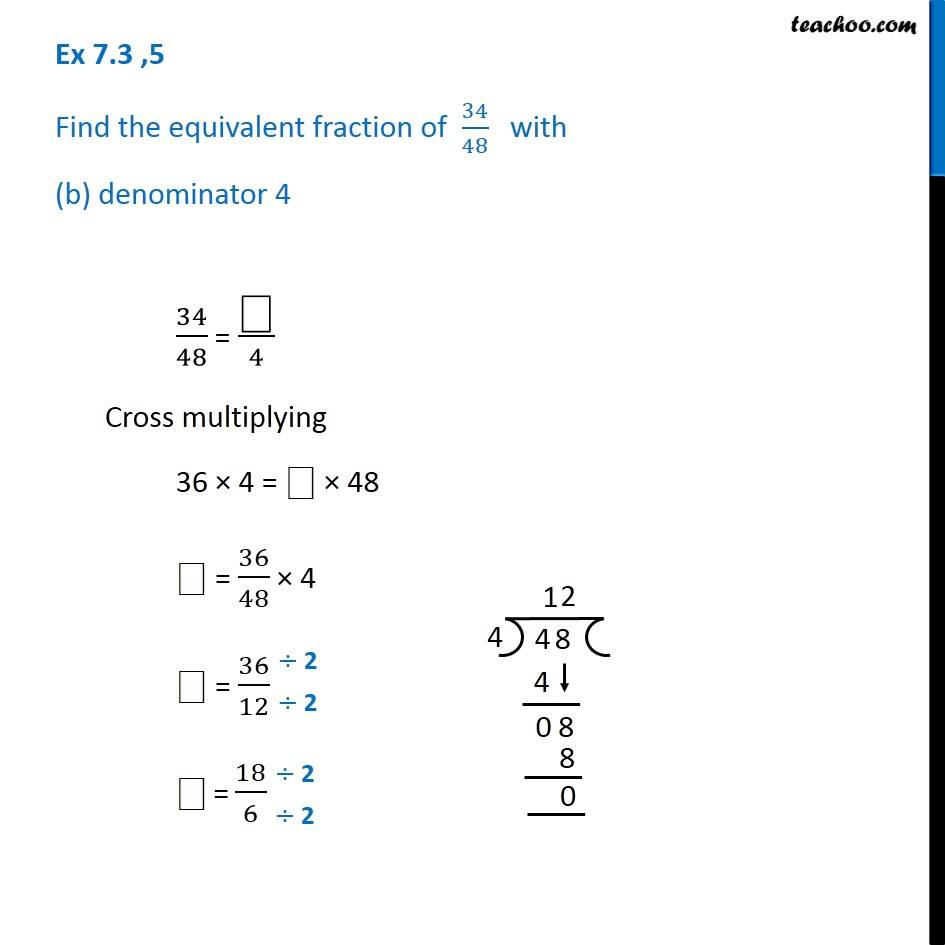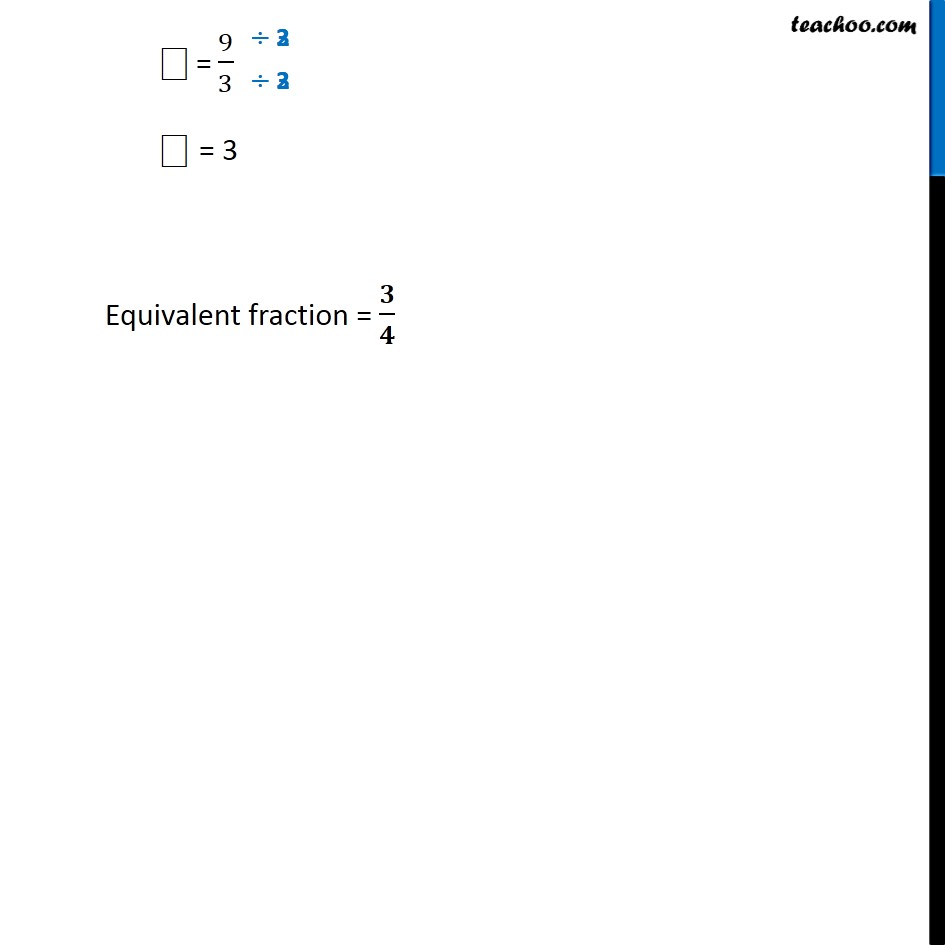Equivalent Fractions

Chapter 7 Class 6 Fractions
Concept wiseIntroducing your new favourite teacher - Teachoo Black, at only ₹83 per month

### Transcript

Ex 7.3 ,5 Find the equivalent fraction of 36/48 with (a) numerator 9 36/48 = 9/"⎕" Cross multiplying 36 × ⎕ = 9 × 48 "⎕" = (9 ×48)/36 "⎕" = (1 × 48)/4 "⎕" = 48/4 "⎕" = 24/2 "⎕" = 12 Equivalent fraction = 𝟗/𝟏𝟐 Ex 7.3 ,5 Find the equivalent fraction of 34/48 with (b) denominator 4 34/48 = "⎕" /4 Cross multiplying 36 × 4 = ⎕ × 48 "⎕" = 36/48 × 4 "⎕" = 36/12 "⎕" = 18/6 "⎕" = 9/3 "⎕" = 3 Equivalent fraction = 𝟑/𝟒